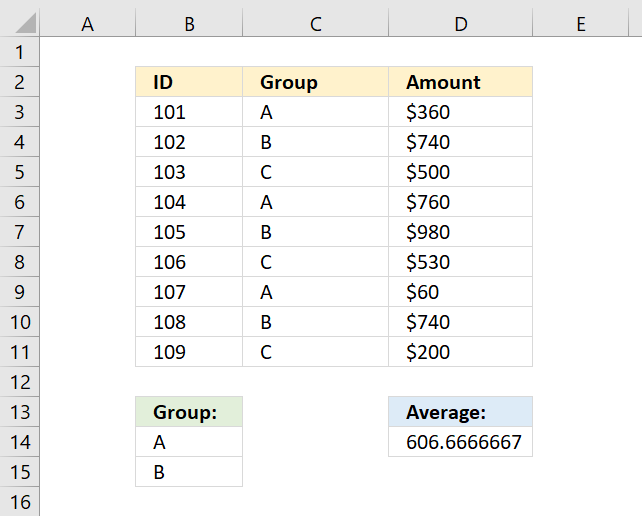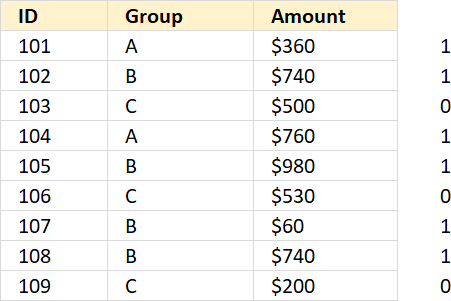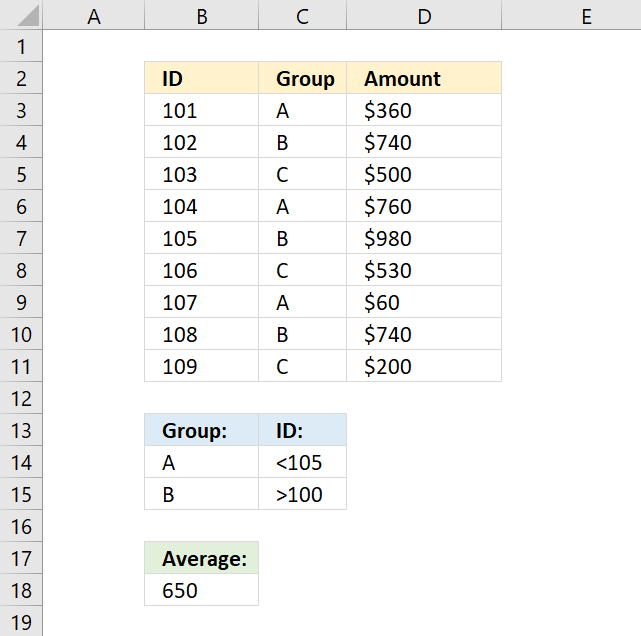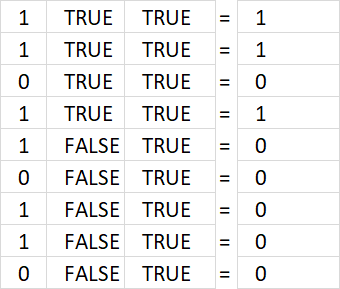Author: Oscar Cronquist Article last updated on December 19, 2018The array formula in cell D14 calculates an average based on multiple criteria in cell range B14:B15. If value in column C is equal to B14 or B15 the amount in column D on the same row is included in the average.

=AVERAGE(IF(COUNTIF(B14:B15,C3:C11),D3:D11,""))

To enter an array formula, type the formula in a cell then press and hold CTRL + SHIFT simultaneously, now press Enter once. Release all keys.

The formula bar now shows the formula with a beginning and ending curly bracket telling you that you entered the formula successfully. Don't enter the curly brackets yourself.

### Explaining formula in cell D14

You can't use AVERAGEIF or AVERAGEIFS function in this example, as far as I know. The COUNTIF function allows you to check if at least one out of multiple values are found in a cell range.

COUNTIF(B14:B15,C3:C11)

Note that I am using multiple values in the second argument, the COUNTIF function returns an array that indicates where group A or B is.

{1;1;0;1;1;0;1;1;0}IF(COUNTIF(B14:B15,C3:C11),D3:D11,"")

The IF function lets you filter values in column D based on COUNTIF function that serves as a logical expression in this case.

IF({1;1;0;1;1;0;1;1;0},D3:D11,"") and returns {360;740;"";760;980;"";60;740;""}

The AVERAGE function then returns the average from the values in the array ignoring the blanks.

AVERAGE({360;740;"";760;980;"";60;740;""})

returns 606.6667 in cell D14.

### AVERAGE - AND OR logicThe following array formula calculates an average based on two conditions, if group is equal to A OR B AND ID is less than 105 AND larger than 100.

=AVERAGE(IF(COUNTIF(B14:B15,C3:C11)*(B3:B11<105)*(B3:B11>100),D3:D11,""))

There are three logical expressions in the first IF argument COUNTIF(B14:B15,C3:C11)*(B3:B11<105)*(B3:B11>100).

The asterisk multiplies the arrays, 1 indicates a location where all three logical tests return TRUE and FALSE if at least one returns FALSE.The array returned from the COUNTIF function is in the first column above, B3:B11<105 is in the second column and B3:B11>100 is in the third column.

The first row: 1*TRUE*TRUE equals 1. All three logical tests are TRUE. The corresponding value is 360 and is included in the AVERAGE calculation.

AVERAGE({360;740;"";760;"";"";"";"";""})

There are two more values that are also in the calculation found in the second and fourth row, 740 and 760.

The average is 620 and is displayed in cell B18.